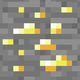# Unresolved Detail In Plotted Equations

Sometimes the calculator detects that an equation is too complicated to plot perfectly in a reasonable amount of time. When this happens, the equation is plotted at lower resolution.

281 out of 311 found this helpful
Have more questions? Submit a request

•\cos\left(x\right)=\sin\left(xy\right)

•Sun Shengkun

\int_a^bf\left(x\right)dx=\sec y

it is lines but have unresolved details

•Sun Shengkun
•Sun Shengkun

\pi x+\cos\left(\frac{\pi x}{5}\right)\left(\sin\ xy\right)\cot\left(x\right)=y

hehe...

•GlitchedGraph666

\sum_{n_1=\sin\left(\sin\left(\sin\left(\sin\left(\sin\left(\sin\left(\sin\left(\sin\left(\sin\left(x!!!!!!!!!\right)\right)\right)\right)\right)\right)\right)\right)\right)}^{x^{x^{x^{x^{x^{\prod_{n_2=y^y}^{y^{2!^{x!!!!!!!!!!!!!!!!!!}}}n_2!!!!!!!!!!!!!!!!!!!^{n_2!!!!!!!}}+x}}}}}n_1^{x^{x^{x^{y^2}}}}+y^{y^{y^{y!!!!!!!!!!!!!!\cdot\prod_{n_{50}=2^{3^{4^{5^{6^{7^x}}}}}}^{\prod_{n_{100}=\prod_{n_{222}=666}^{x!!!!!!!!!!!!!}\left(n_{222}!^{n_{222}!^{n_{222}!}}\right)}^{\tan\left(y+\tan\left(y+\tan\left(y+\tan\left(y+\tan\left(y+\tan\left(y+\tan\left(y+\tan\left(y+\tan\left(y+\tan\left(y+\tan\left(y+x\right)\right)\right)\right)\right)\right)\right)\right)\right)\right)\right)}n_{100}!!!!!!!!}n_{50}!!!!!!!!!!}}}>x!!!!!!!!!!!!!!!!!!!!!!!!!!!!!!!!!!!!!!!!!!!!!!!!+y!!!!!!!!!!!!!!!!!!!!!!!!!!!!!!!!!!+\prod_{n_{732}=y!!!}^{x!!!}\sin\left(x!!!^{n_{732}!^{^{n_{732}!^{^{n_{732}!^{^{n_{732}!^{^{n_{732}!^{666}}}}}}}}}}\right)

•Charliedevelin

x!!!!!!!!!!!!!!!=y!!!!!!!!!!

•Andrew Carratu

and this:

\csc\left(\pi x+\cos\left(\frac{\pi x}{5}\right)\left(\sin\ xy\right)\cot\left(x\right)\right)=\sec\left(y\right)

•Orion D. Hunter

sin(x!)=sin(y!) and x!=y! actually behaves like x=y but it has a difference in desmos

•Orion D. Hunter

https://www.desmos.com/calculator/spjy78xgeb just, why, was, sin(x!!)=sin(y!!) not, plotted, like, X=Y

•Tanksear Industries
•Thecubicalguy1
•Joshua Lewinbl

mod(x,sin(xy)^2)=y

•168673
•Lie

This one is laggy so beware! \frac{x}{y}=\frac{3}{\sec\left(x^{5^y}\right)}

•Lie

This is not that detailed but I thought it looked SO COOL! \sqrt{x^2y^2}=\frac{9}{\sec\left(\pi x\right)}

•Lie

Put this in radian mode it looks cool: y=\frac{\cot\left(x\right)}{\tan\left(y\right)}

•Lie
•Orion D. Hunter
Edited by Orion D. Hunter
•Orion D. Hunter

•Lie

(but it has to be in radians degrees is too anticlimactic): x^y=9\sin\left(\cot\left(3x\right)\right)

•Filip Sedláček

2^x * 2^y=2^(x+y)

•D35M05BR34K4G3MA5T3R

How about some crazy art?  To see it, go to https://www.desmos.com/calculator/z5o7ikzpwu .

•Daemon Morrisoneagan

this never ends. no matter how far you zoom, the is an endless pattern. \log\left(\tan\left(\cos\left(\ln x^2\right)\right)\right)=\log\left(\cot\left(\sin\left(\ln y^2\right)\right)\right)

and this one i just can't explain.                                                                                        \log\left(\tan\left(\cos\left(\left(\ln x^2\right)!!\right)\right)\right)=\log\left(\cot\left(\sin\left(\left(\ln y^2\right)!!\right)\right)\right)

Edited by Daemon Morrisoneagan
•Tanksear Industries

\sin\left(x-\sqrt[x-y^{\frac{\sqrt{\sqrt{xy^{x^{\frac{y}{x^y}}}-x^{yx}}}}{\sqrt{x\sqrt{x\sqrt{y\sqrt{\sqrt{x}}}}}}}]{\sin\left(\sqrt{x-\frac{\sin\left(x\right)}{x-y}}\right)}\left|\sin\left(\sqrt{x}\right)\right|\right)\le\sin\left(\sqrt{\sqrt{x^y}\sqrt{y-x^{yxy}}}\right)

•Daemon Morrisoneagan
•ANDREW TUCKER

\tan\left(\frac{\sin\left(x+\tan\left(y\right)\right)}{y!-\sin\left(x\right)}\right)=\tan\left(\frac{\tan\left(y+\sin\left(x\right)\right)}{x!-\tan\left(y\right)}\right)

\sin\left(x!^{\log\left(y!\right)}\right)=\tan\left(y^{\log\left(x\right)}\right)

y=\tan\left(\frac{\sin\left(\tan\left(\frac{\tan\left(\frac{\sin\left(\frac{\sin\left(\tan\left(x\right)\right)}{\cos\left(\sin\left(y\right)\right)}\right)}{y-\tan\left(x\right)}\right)}{\tan\left(\tan\left(\frac{\tan\left(\tan\left(x\right)\right)}{y}\right)\right)}\right)\right)}{\tan\left(\tan\left(\tan\left(\frac{\tan\left(\sin\left(x\right)\right)}{y+\tan\left(x\right)}\right)\right)\right)}\right)

y=\tan\left(\frac{\tan\left(\frac{\sin\left(x+\tan\left(x\right)\right)}{y}\right)}{\sin\left(\tan\left(y\right)\right)}\right)

•Carson Perkins

y=tan(cos(x^5(y)))

•ANDREW TUCKER

\tan\left(\frac{\tan\left(\sin\left(x\right)\right)\sin\left(\log\left(y\right)\right)}{\sin\left(x!-\cos\left(y\right)\right)!}\right)=\cos\left(\sqrt{\sin\left(\frac{\tan\left(x+\sin\left(y!\right)\right)}{x\cos\left(y-\tan\left(\sin\left(x!\right)\right)\right)}\right)!}\right)

This one is a mess

https://www.desmos.com/calculator/hzyizn3wep

•Composer Machine

\tan\left(2\cdot\cos\left(x\right)\right)=\frac{\sin\left(y^2-x^2\right)}{\log\left(2\right)}

this makes an interesting design although it seems to form into a 'straighter line' as you zoom out on the left and right sides!

•Yang Pan

I was just bored
lol
https://www.desmos.com/calculator/uev2f3dwmw

This one does the opposite of what others do. The more you zoom out, the more it becomes focused. Until, it just stops loading.

Edited by Yang Pan